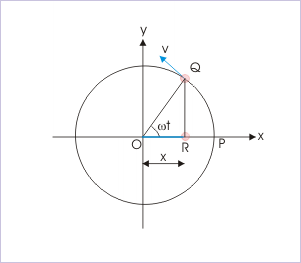# Oscillations - Online Test

Q1. The pendulum of a wall clock executes
Explaination / Solution:

It executes simple harmonic motion because in this motion restoring force is directly proportional to the displacement towards the mean position at any time.

Q2. Oscillatory motion is best described by
Explaination / Solution:

In oscillatory motion object moves to and fro about mean position periodically or after a particular interval of time.All oscillatory motions are periodic in nature.

Q3. How can you describe a periodic motion
Explaination / Solution:

A motion of a body that repeats itself at regular intervals of time on the same path like rotation of earth around sun in 365.25 days

Q4. Period of a periodic motion is the ________ interval of time after which the motion is __________.
Explaination / Solution:

Time period of an oscillatory motion is the smallest interval of time for one complete oscillation.As when we apply the force on the bob,bob moves from mean position to extreme position then form extreme position to mean position and then again from mean to extreme in opposite direction and at last come to mean position.The time taken by bob in this whole process is called period of oscillation.

Q5. Frequency of the periodic motion is
Explaination / Solution:

Frequency is defined as number of oscillation completed by an oscillating body in unit time i.e. in one second during oscillatory motion.

Q6. for any periodic motion with a period T and displacement = f(t)
Explaination / Solution:
No Explaination.

Q7. Any periodic function can be expressed as superposition of-
Explaination / Solution:

Because sine and cosine functions both are periodic in nature and according to Fourier theorem a" Fourier series" is a way to represent a function as the sum of simple sine waves. More formally, it decomposes any periodic function or periodic signal into the sum of a (possibly infinite) set of simple oscillating functions, namely sines and cosines, or in simple terms any periodic function can be expressed as sum or superposition of sine and cosine functions with fixed time periods.

Q8. Simple harmonic motion is motion represented by
Explaination / Solution:

Equation of simple harmonic motion is given by

Where x(t) is the displacement of body at any instant of time

A is the amplitude of the body

is the phase angle at the instant of time.

Q9. Simple harmonic motion is the projection of _____________________ of the circle in which the latter motion takes place.
Explaination / Solution:Simple harmonic motion is the projection of uniform circular motion.In the above diagram P is the position of particle at at any instant of time.This motion follows the condition of SHM.simple harmonic motion is a type of periodic motion or oscillation motion where the restoring force is directly proportional to the displacement and acts in the direction opposite to that of displacement

Q10. The total mechanical energy of a harmonic oscillator
Explaination / Solution:
No Explaination.